# 磁盘iops 测试 fio 及报错解释

fio使用：http://elf8848.iteye.com/blog/2168876       后面有各参数对应的解释  以及 多个job时 写在job file里面

I/O输入/输出(Input/Output)，读和写，提高缓存(cache)和做磁盘阵列(RAID)能提高存储IO性能。
IOPS (英文：Input/Output Operations Per Second)，即每秒进行读写（I/O）操作的次数，多用于数据库、存储等场合，衡量随机访问的性能。
存储端的IOPS性能和主机端的IO是不同的，IOPS是指存储每秒可接受多少次主机发出的访问主机的一次IO需要三次访问存储才可以完成例如，主机写入一个最小的数据块，也要经过“发送写入请求、写入数据、收到写入确认”等三个步骤，也就是3个存储端访问。
Linux下常用Fio、dd工具，  Windows下常用IOMeter。
FIO是测试IOPS的非常好的工具，用来对硬件进行压力测试和验证，支持13种不同的I/O引擎，包括:sync,mmap, libaio, posixaio, SG v3, splice, null, network, syslet, guasi, solarisaio 等等。
注意：不要对有数据的磁盘或者分区做测试，会破坏已存在的数据

fio -filename=/dev/sdb1 -direct=1 -iodepth 1 -thread -rw=randread -ioengine=psync -bs=4k -size=50G -numjobs=10 -runtime=1000 -group_reporting -name=mytest

filename=/dev/sdb1     测试文件名称，通常选择需要测试的盘的data目录。 只能是分区，不能是目录，会破坏数据。
direct=1                     测试过程绕过机器自带的buffer。使测试结果更真实。
iodepth 1                   队列深度，只有使用libaio时才有意义，这是一个可以影响IOPS的参数，通常情况下为1。
rw=randwrite              测试随机写的I/O
rw=randrw                测试随机写和读的I/O
ioengine=psync           io引擎使用pync方式
bs=4k                   单次io的块文件大小为4k

bsrange=512-2048         同上，提定数据块的大小范围
size=50G    本次的测试文件大小为50g，以每次4k的io进行测试,此大小不能超过filename的大小，否则会报错。
numjobs=10               本次的测试线程为10.
runtime=1000             测试时间为1000秒，如果不写则一直将5g文件分4k每次写完为止。
rwmixwrite=30            在混合读写的模式下，写占30%

group_reporting          关于显示结果的，汇总每个进程的信息。

lockmem=1g               只使用1g内存进行测试。
zero_buffers             用0初始化系统buffer。
nrfiles=8                每个进程生成文件的数量。

write 顺序写

randwrite 随机写

randrw 随机混合读写

fio -filename=/dev/sdb1 -direct=1 -iodepth 1 -thread -rw=read -ioengine=psync -bs=4k -size=50G -numjobs=30 -runtime=1000 -group_reporting -name=mytest

fio -filename=/dev/sdb1 -direct=1 -iodepth 1 -thread -rw=randwrite -ioengine=psync -bs=4k -size=50G -numjobs=30 -runtime=1000 -group_reporting -name=mytest

fio -filename=/dev/sdb1 -direct=1 -iodepth 1 -thread -rw=write -ioengine=psync -bs=4k -size=50G -numjobs=30 -runtime=1000 -group_reporting -name=mytest

fio -filename=/dev/sdb1 -direct=1 -iodepth 1 -thread -rw=randrw -rwmixread=70 -ioengine=psync -bs=4k -size=50G -numjobs=30 -runtime=100 -group_reporting -name=mytest -ioscheduler=noop

三，实际测试范例：

[root@localhost ~]# fio -filename=/dev/sdb1 -direct=1 -iodepth 1 -thread -rw=randrw -rwmixread=70 -ioengine=psync -bs=16k -size=200G -numjobs=30 -runtime=100 -group_reporting -name=mytest1              //因为200G，超过了/dev/sdb1的大小，所以机器重启后，报错起不了。

mytest1: (g=0): rw=randrw, bs=16K-16K/16K-16K, ioengine=psync, iodepth=1
mytest1: (g=0): rw=randrw, bs=16K-16K/16K-16K, ioengine=psync, iodepth=1
fio 2.0.7
Jobs: 1 (f=1): [________________m_____________] [3.5% done] [6935K/3116K /s] [423 /190  iops] [eta 48m:20s] s]
mytest1: (groupid=0, jobs=30): err= 0: pid=23802                                       // iops：磁盘的每秒读写次数，这个是随机读写考察的重点
read : io=1853.4MB, bw=18967KB/s, iops=1185 , runt=100058msec   //bw：磁盘的吞吐量，这个是顺序读写考察的重点，类似于下载速度
clat (usec): min=60 , max=871116 , avg=25227.91, stdev=31653.46   //avg每个IO请求的平均相应时间25秒左右
lat (usec): min=60 , max=871117 , avg=25228.08, stdev=31653.46
clat percentiles (msec):
|  1.00th=[    3],  5.00th=[    5], 10.00th=[    6], 20.00th=[    8],
| 30.00th=[   10], 40.00th=[   12], 50.00th=[   15], 60.00th=[   19],
| 70.00th=[   26], 80.00th=[   37], 90.00th=[   57], 95.00th=[   79],
| 99.00th=[  151], 99.50th=[  202], 99.90th=[  338], 99.95th=[  383],    // %99.95的IO请求的响应时间是小于等于383msec
| 99.99th=[  523]
bw (KB/s)  : min=   26, max= 1944, per=3.36%, avg=636.84, stdev=189.15
write: io=803600KB, bw=8031.4KB/s, iops=501 , runt=100058msec
clat (usec): min=52 , max=9302 , avg=146.25, stdev=299.17
lat (usec): min=52 , max=9303 , avg=147.19, stdev=299.17
clat percentiles (usec):
|  1.00th=[   62],  5.00th=[   65], 10.00th=[   68], 20.00th=[   74],
| 30.00th=[   84], 40.00th=[   87], 50.00th=[   89], 60.00th=[   90],
| 70.00th=[   92], 80.00th=[   97], 90.00th=[  120], 95.00th=[  370],
| 99.00th=[ 1688], 99.50th=[ 2128], 99.90th=[ 3088], 99.95th=[ 3696],
| 99.99th=[ 5216]
bw (KB/s)  : min=   20, max= 1117, per=3.37%, avg=270.27, stdev=133.27
lat (usec) : 100=24.32%, 250=3.83%, 500=0.33%, 750=0.28%, 1000=0.27%
lat (msec) : 2=0.64%, 4=3.08%, 10=20.67%, 20=19.90%, 50=17.91%
lat (msec) : 100=6.87%, 250=1.70%, 500=0.19%, 750=0.01%, 1000=0.01%
cpu          : usr=1.70%, sys=2.41%, ctx=5237835, majf=0, minf=6344162
IO depths    : 1=100.0%, 2=0.0%, 4=0.0%, 8=0.0%, 16=0.0%, 32=0.0%, >=64=0.0%
submit    : 0=0.0%, 4=100.0%, 8=0.0%, 16=0.0%, 32=0.0%, 64=0.0%, >=64=0.0%
complete  : 0=0.0%, 4=100.0%, 8=0.0%, 16=0.0%, 32=0.0%, 64=0.0%, >=64=0.0%
issued    : total=r=118612/w=50225/d=0, short=r=0/w=0/d=0

Run status group 0 (all jobs):
READ: io=1853.4MB, aggrb=18966KB/s, minb=18966KB/s, maxb=18966KB/s, mint=100058msec, maxt=100058msec
WRITE: io=803600KB, aggrb=8031KB/s, minb=8031KB/s, maxb=8031KB/s, mint=100058msec, maxt=100058msec

sdb: ios=118610/50224, merge=0/0, ticks=2991317/6860, in_queue=2998169, util=99.77%    //利用率达到99.77%

bw：磁盘的吞吐量，这个是顺序读写考察的重点，类似于下载速度。
iops：磁盘的每秒读写次数，这个是随机读写考察的重点

io总的输入输出量

runt：总运行时间

lat (msec)：延迟(毫秒)

msec： 毫秒
usec： 微秒

$fio --name=global --direct=1 --name=read --rw=read --size=10m --name=write --rw=randwrite --size=5m 与上述命令行选项具有相同意义的配置文件如下所示。 # cat fio_exam.fio //文件名 [global] direct=1 [readjob] size=10m rw=read [writejob] size=5m rw=randwrite 使用这个配置文件执行fio命令如下。$ fio simple_read.fio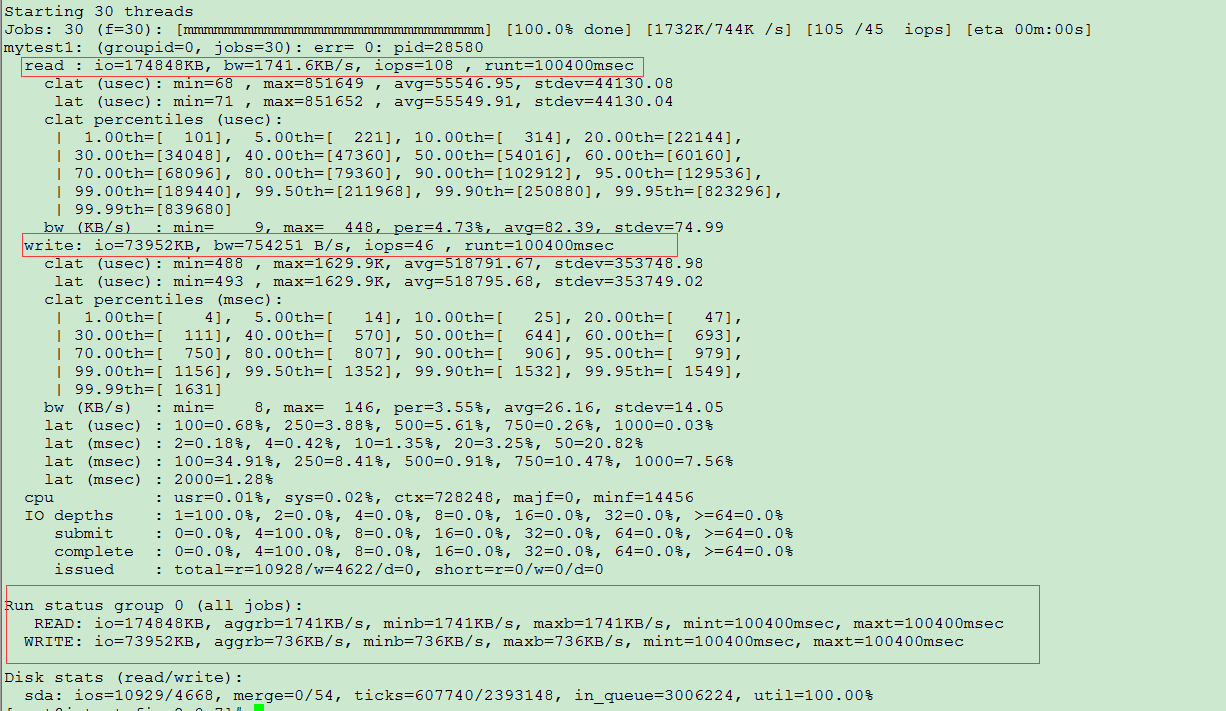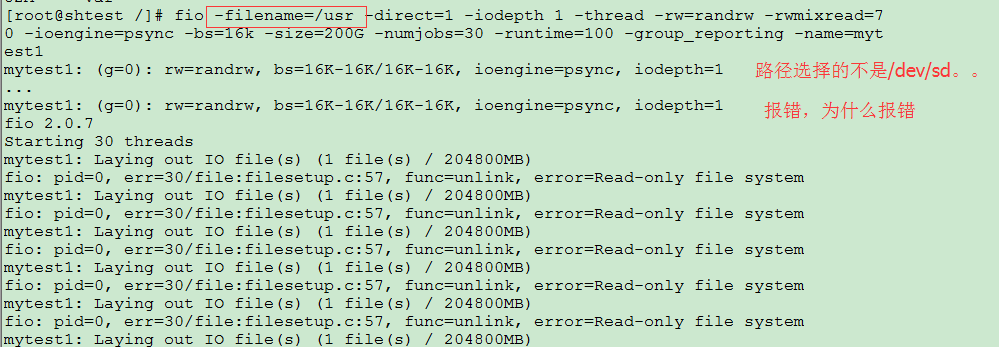iodepth 队列深度对IOPS的影响 :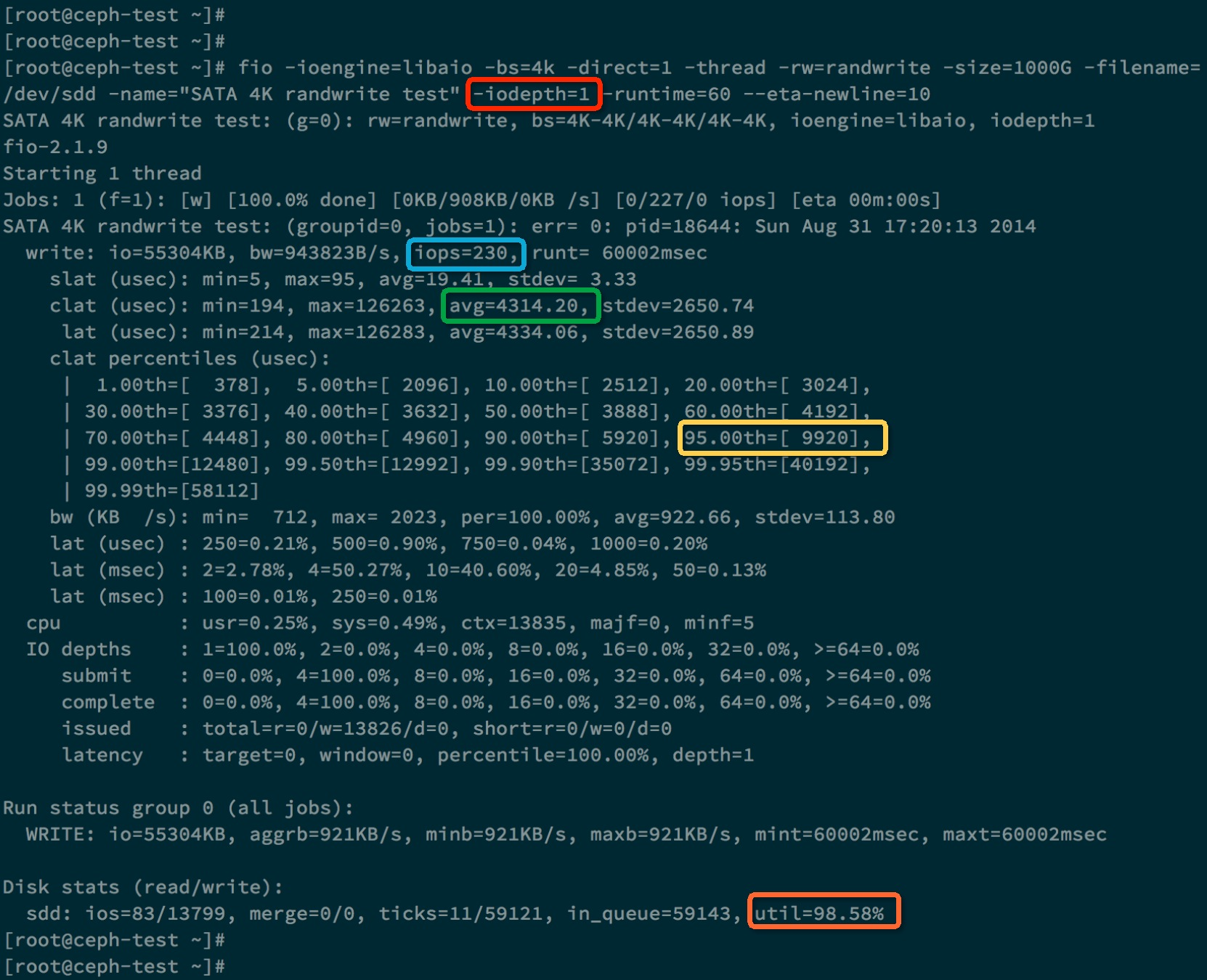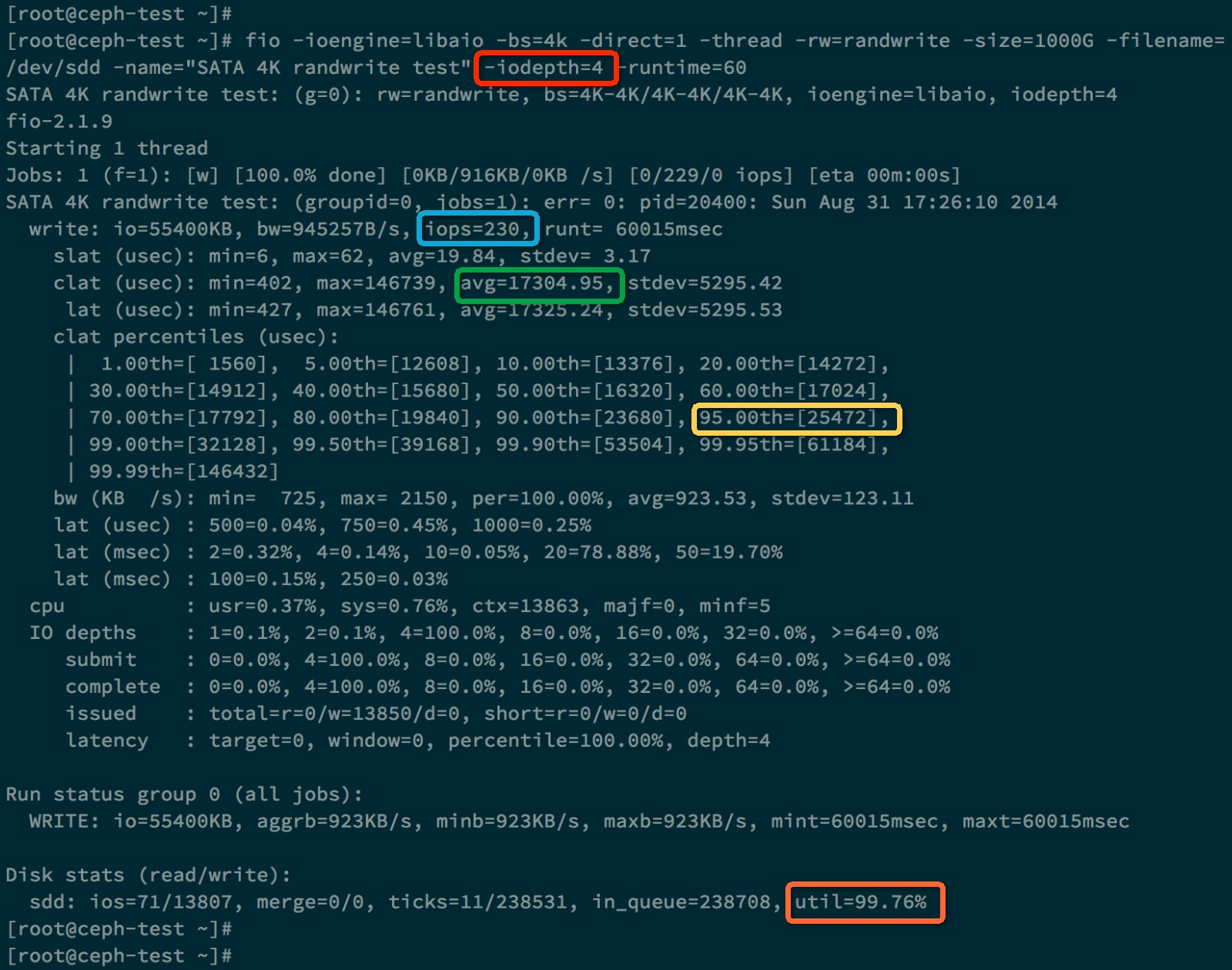## 寻址空间对IOPS的影响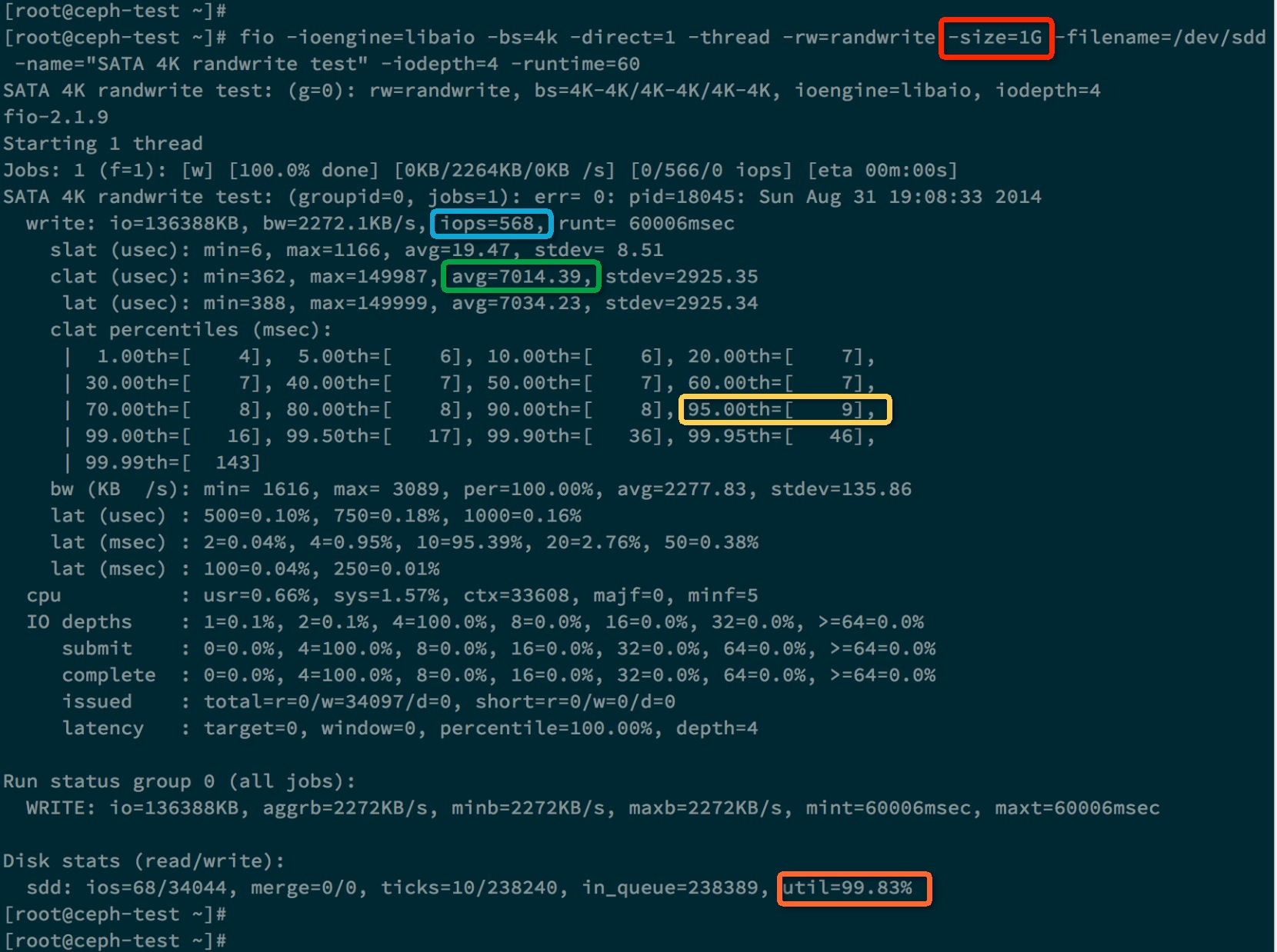## 硬盘优化

• 提高转速(降低旋转时间和传输时间)
• 增加Cache(降低写延迟，但不会提高IOPS)
• 提高单磁道密度(变相提高传输时间)

# RAID测试

RAID0/RAID5/RAID6的多块磁盘可以同时服务，其实就是提高并行度，这样极大提高了性能(相当于银行有多个柜台)。

# 根据RAID和磁盘类型磁盘IOPS的计算

1、RAID类型的读写比

 RAID类型 公式 RAID5、RAID3 Drive IOPS=Read IOPS + 4*Write IOPS RAID6 Drive IOPS=Read IOPS +  6*Write IOPS RAID1、RAID10 Drive IOPS=Read IOPS + 2*Write IOPS

2、硬盘类型的IOPS值

 硬盘类型 IOPS FC 15K RPM 180 FC 10K RPM 140 SAS 15K RPM 180 SAS 10K RPM 150 SATA 10K RPM 290 SATA 7.2K RPM 80 SATA 5.4K RPM 40 Flash drive 2500

3、具体业务系统的读写比

1) 业务需求： 10TB 的FC 15K RPM存储空间，满足6000 IOPS，计算RAID5RAID10分别需要多少块硬盘？

不同的RAID类型Drive 硬盘实际IOPS负载分别如下：

RAID10：（2/3*6000+2*1/3*6000= 8000 IOPS

RAID5：（2/3*6000+4*1/3*6000=12000 IOPS

RAID108000 /180 = 45

RAID512000/180 =67

2)   一个RAID5，是由5500G 10K RPMFC盘组成，换算出该RAID支持的最大IOPS以及能够给前端应用提供的IOPS？

2/3*X+4*1/3*X = 700

2*X = 700

X=350

能够提供给前端应用的IOPS350

11-21107

#### fio造成内核崩溃01-112万+

#### Fio 输出内容的解释

02-20117

#### linux 使用FIO测试磁盘iops

06-221266

#### linux编译安装fio(支持rbd引擎)

06-264320

#### FIO使用说明©️2020 CSDN 皮肤主题: 大白 设计师: CSDN官方博客点击重新获取扫码支付1.余额是钱包充值的虚拟货币，按照1:1的比例进行支付金额的抵扣。
2.余额无法直接购买下载，可以购买VIP、C币套餐、付费专栏及课程。余额充值